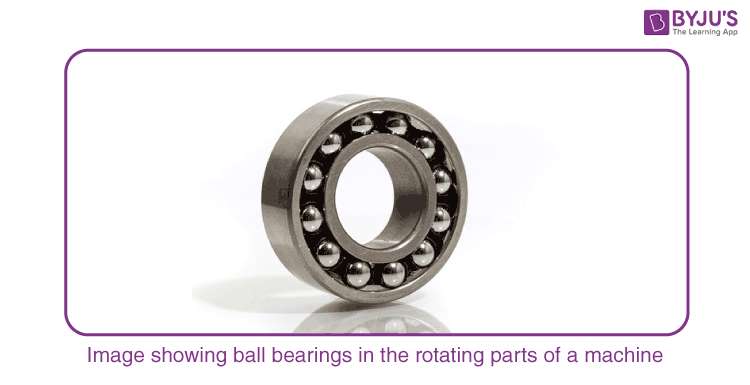Jet Set Go! All about Aeroplanes Jet Set Go! All about Aeroplanes

# Rolling Friction And Rolling Resistance

You must have noticed that it is very difficult to pull a heavy almirah on a rough floor. But, if you attach four iron wheels to the almirah, then it becomes much easier to pull it. The iron wheel or in fact any wheel does not slide on the floor. Wheels roll on the floor. This means that the surface of the wheel in touch with the ground doesn’t rub against the ground or slide on it. The velocity of the point of contact of the wheel with respect to the floor remains zero, and yet, the wheel keeps moving forward. Let’s discuss the Rolling friction to understand well.

## What is a Rolling Friction?

It is the force that resists or slows down the motion of a wheel or a ball (the curved surface) rolling on the ground. When one body is said to be rolling on another body or surface, ideally it is assumed that there is no slipping or sliding. Rolling friction is a weak force, which explains the reason why it is easier to pull an almirah with wheels in it. It is so weak that the magnitude of rolling friction is negligible as compared to static, kinetic, or limiting friction. Rolling friction is often a combination of several types of frictions acting between the point of contact of the curved surface and the ground.

Notice that it is not always necessary that a wheel or a sphere will roll. It may slide depending upon its motion, and the forces acting on it. Sometimes, to reduce the energy losses due to friction, small steel balls are included in the rotating parts of the wheel machines which are known as ball bearings.### Rolling Friction Formula

The general equation to calculate the rolling friction is given by the expression:

$$\begin{array}{l}F_r=\mu_rN\end{array}$$
where,
F is the rolling friction
µr is the coefficient of rolling friction
N is the normal reaction on the object

### Factors effecting rolling friction

Following are the factors affecting the rolling friction

• The shape of the wheel or the curved surface
• Nature of the surface on which it is rolling
• Speed of the wheel
• The radius of the wheel
• Nature of the material of the wheel or the curved surface

## Rolling Friction Examples

Following are a few examples of rolling friction

• When the surface of the rolling object is soft, due to physical and other material considerations the surface of the object in contact gets deformed which in turn results in the increase of the rolling friction. It is due to this reason why it is hard to ride a bicycle with a flat tire.
• If it is the other way round, i.e., if the surface on which the object rolls is soft then we witness the ploughing action. This situation is experienced when a vehicle is driven on muddy surfaces.

## Frequently Asked Questions – FAQs

### Define rolling friction.

It is the force that resists or slows down the motion of the curved surface, like a wheel or a ball rolling on the ground is known as rolling friction.

### What is the general equation to calculate the rolling friction?

$$\begin{array}{l}F_r=\mu_rN\end{array}$$

### What are the three factors affecting the rolling friction?

• Speed of the wheel.
• The radius of the wheel.
• The shape of the wheel or the curved surface.

True.

### Does the nature of the material of the wheel or the curved surface affect the rolling friction?

Yes, the nature of the material of the wheel or the curved surface affects the rolling friction.
Test your knowledge on Rolling Friction And Rolling Resistance